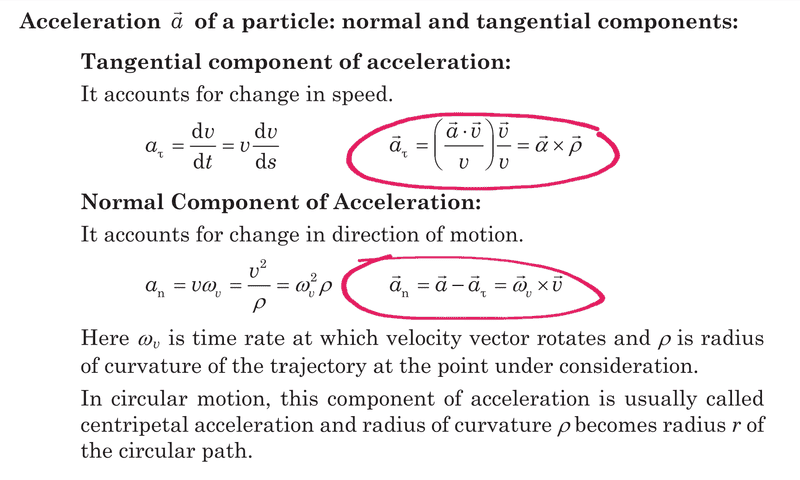# Tangential & Normal acceleration in Circular Motion

Shreya
Homework Statement:
Can I get an intuition (or a derivation) of the equations in the coloured boxes. Please refer the image.
Relevant Equations:Homework Helper
Gold Member
2022 Award
•Shreya
Homework Helper
Gold Member
2022 Award
The tangential acceleration is the component of the acceleration that is parallel to the velocity.
For any two vectors ##\frac{\vec x.\vec y}{|\vec y|}## is the magnitude of the component of ##\vec x## parallel to ##\vec y## , and ##\frac{\vec y}{|\vec y|}## is the unit vector parallel to ##\vec y##, so the product of the two ##\frac{\vec x.\vec y}{|\vec y|}\frac{\vec y}{|\vec y|}## is the component of ##\vec x## parallel to ##\vec y##. That yields the first part of the first circled equation.

The normal (or centripetal) acceleration is the component normal to the velocity, the tangential and normal accelerations add vectorially to give the whole acceleration. I'll have to think more on how to view the vector equation for that.

•Shreya
Homework Helper
Gold Member
Given any vector ##\vec A## and any vector ##\vec V##,
one can write ##\vec A## as the sum of two vectors:
one parallel to ##\vec V## and the other (the rest of ##\vec A##) perpendicular to ##\vec V##.

\begin{align*}
\vec A
&=\vec A_{||V} + (\vec A - \vec A_{||V})
\end{align*}

Now,
\begin{align*}
\vec A_{||V}
&= (\vec A \cdot \hat V) \hat V\\
&= (\vec A \cdot \frac{\vec V}{V}) \frac{\vec V}{V}
\end{align*}
[As a special case, let $\hat V = \hat x$.]
(Check that $\vec A_{||V}\cdot \hat V=(\vec A \cdot \hat V)$
and that $(\vec A - \vec A_{||V})\cdot \hat V=0$.)

For a trajectory, $\hat V$ is tangent to the trajectory and (for a planar curve)
$\hat V_{\bot}$ is normal to the trajectory.

So, if $\vec A$ is the acceleration vector ($\vec A =\frac{d}{dt}\vec V$),
then $\vec A_{||V}$ is the tangential-part of the acceleration vector (responsible for changing the magnitude of $\vec V$ [i.e. speeding up or slowing down]),
and the other part is the normal-part of the acceleration vector (responsible for changing the direction of $\vec V$ [turning the velocity vector] ).

Next, one has to express these in terms of the angular pseudovectors $\vec \alpha$ and $\vec \omega$ [probably should use a different "arrowhead"] (which when crossed with ordinary [polar] vectors result in ordinary [polar] vectors).

You'll probably [implicitly] use the "BAC-CAB" rule https://en.wikipedia.org/wiki/Triple_product#Vector_triple_product
https://mathworld.wolfram.com/BAC-CABIdentity.html

Last edited:
•Shreya and PeroK
Shreya
The tangential acceleration is the component of the acceleration that is parallel to the velocity.
That makes sense now. Thanks haruspex. About the second part of 1st equation: I think they are multiplying the perpendicular component of angular acceleration with radius vector giving dv/dt.

Shreya
pseudovectors
What is meant by pseudovectors?

Homework Helper
Gold Member
2022 Award
•Shreya
Shreya
Everything is online these days:
True, thanks a lot for the link though!

Shreya
I think I get both the equations now! Thanks a lot PeroK, robphy and Haruspex! I really appreciate your help.

•